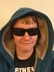# Wills Flash Cards- GeomentryQuestion 1: What is the area of a circle with a diameter of 16?

Question 2: The circumference of a circle is 30pi?

Question 3: What is the sum of measures of the interior angle of a hexagon?

Question 4: If a circle has the diameter of 8 what is the circumference?

Question 5: 1,222 x 3 =